# Tree examples for assignment 4

This page has some examples of trees built using the Branch and Leaf constructors specified in assignment 4.

{-# LANGUAGE FlexibleContexts #-}
module A04Trees where
import A04Sol hiding (main)
import System.IO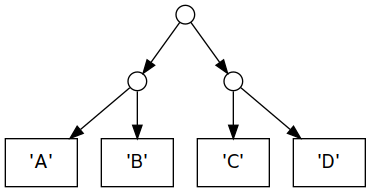balancedCharTree1 :: Tree Char
balancedCharTree1 =
Branch (Branch (Leaf 'A') (Leaf 'B'))
(Branch (Leaf 'C') (Leaf 'D'))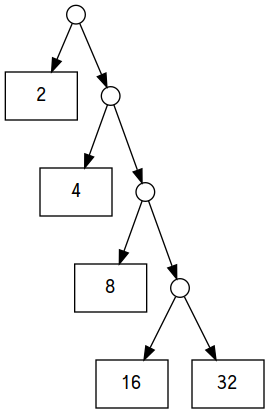rightSkewedIntTree1 :: Tree Int
rightSkewedIntTree1 =
Branch (Leaf 2)
(Branch (Leaf 4)
(Branch (Leaf 8)
(Branch (Leaf 16)
(Leaf 32))))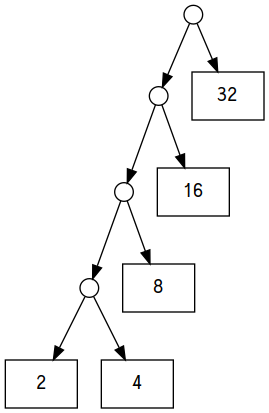leftSkewedIntTree1 :: Tree Int
leftSkewedIntTree1 =
Branch (Branch (Branch (Branch (Leaf 2)
(Leaf 4))
(Leaf 8))
(Leaf 16))
(Leaf 32)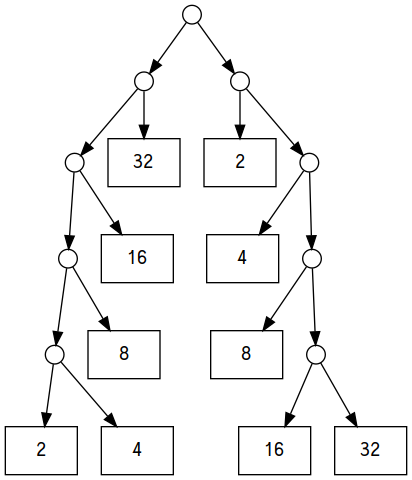pairOfTrees :: Tree Int
pairOfTrees = Branch leftSkewedIntTree1 rightSkewedIntTree1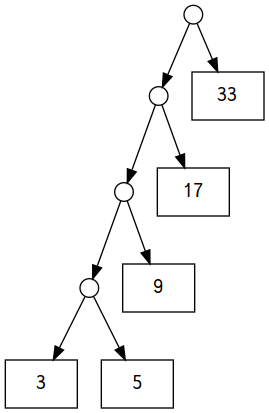This is the result of applying succ to each leaf of the previous tree.

leftSkewedIntTree2 :: Tree Int
leftSkewedIntTree2 = treeMap succ leftSkewedIntTree1This is a tree containing some strings.

stringTree1 :: Tree String
stringTree1 =
Branch (Branch (Leaf "ant")
(Branch (Leaf "bee")
(Leaf "cat")))
(Branch (Leaf "dog")
(Leaf "eel"))

# Generating figures

You don’t necessarily need to look at this section, but I’ll describe what it does in case you’re curious. The function graphviz takes a tree and converts it into a string in a language called GraphViz that can then be fed to a command-line tool to produce images. All the diagrams of trees on this page were produced from this Haskell program and GraphViz.

graphviz :: Show a => Tree a -> String
graphviz tree = surround $snd$ execRWS (traverse tree) () 0
where
surround s = "digraph {\n" ++ s ++ "}\n"
quote a = "\"" ++ concatMap subst (show a) ++ "\""
subst '"' = "\\\""
subst c = [c]
increment :: MonadState Int m => (Int -> m a) -> m Int
increment f = do
i <- get
put (i+1)
f i
return i
node i = "node" ++ show i
traverse (Leaf x) = increment $\i -> tell$ node i ++ " [shape=rectangle, label=" ++ quote x ++ "]\n"
traverse (Branch left right) = increment $\i -> do j <- traverse left k <- traverse right tell$
node i ++ " [shape=circle, width=0.2, label=\"\"]\n" ++
node i ++ " -> " ++ node j ++ "\n" ++
node i ++ " -> " ++ node k ++ "\n"
createGraph :: (String, String) -> IO ()
createGraph (name, dot) =
putStrLn ("Creating " <> filename) >>
withFile filename WriteMode (flip hPutStr dot)
where
filename = "a04" ++ name ++ ".dot"
main :: IO ()
main = mapM_ createGraph
[ ("bctree1", graphviz balancedCharTree1)
, ("ritree1", graphviz rightSkewedIntTree1)
, ("litree1", graphviz leftSkewedIntTree1)
, ("litree2", graphviz leftSkewedIntTree2)
, ("stree1", graphviz stringTree1)
, ("pair", graphviz pairOfTrees)
]

Used in some tests:

square x = x*x